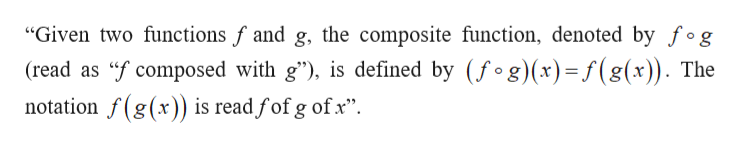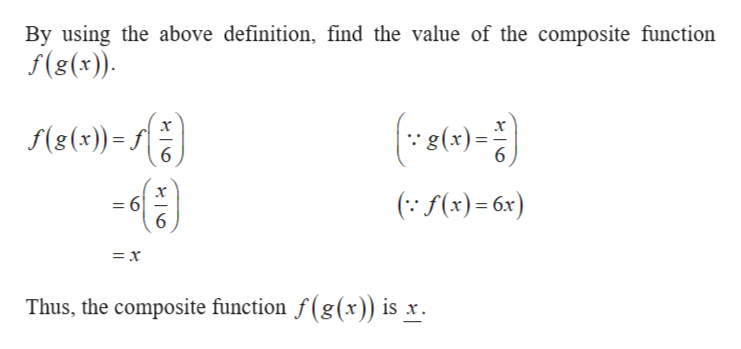Find f(g(x)) and g(f(x)) and determine whether the pair of functions f and g are inverses of each other.Xf(x) 6x and g(x)f(g(x)-a.b. g(fx)C.O fand g are not inverses of each otherO fand g are inverses of each other.Click to select your answer(s).earchhpHEWLE T T - P AC KARD

Questionhelp_outlineImage TranscriptioncloseFind f(g(x)) and g(f(x)) and determine whether the pair of functions f and g are inverses of each other. X f(x) 6x and g(x) f(g(x)- a. b. g(fx) C. O fand g are not inverses of each other O fand g are inverses of each other. Click to select your answer(s). earch hp HEWLE T T - P AC KARD fullscreen
Step 1

The given functions are,

Step 2

Definition used:help_outlineImage Transcriptionclose"Given two functions f and g, the composite function, denoted by fog (read as f composed with g"), is defined by (fog)(x)= f(g(x)). The notation f(g(x)) is read fof g ofx" fullscreen
Step 3

a) Obtain the composition function &l...help_outlineImage TranscriptioncloseBy using the above definition, find the value of the composite function f(g(x)) f(g(x))- 6 g(x)= (f(x)6x) =6 6 - =x Thus, the composite function f(g(x)) is x fullscreen

Want to see the full answer?

See Solution

Want to see this answer and more?

Our solutions are written by experts, many with advanced degrees, and available 24/7

See Solution
Tagged in

Other